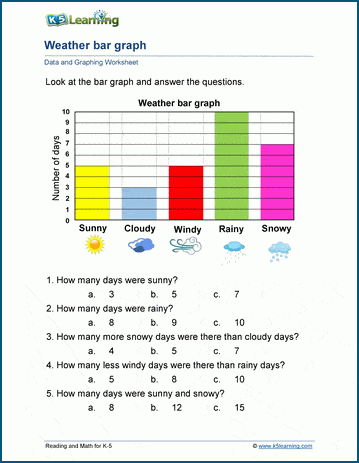# Data & Graphing Worksheets

## Collecting and analyzing data

Our data and graphing worksheets include data collection, tallies, pictographs, bar charts, line plots, line graphs, circle graphs, venn diagrams and introductory probability and statistics.

Kindergarten graphing worksheets

Grade 1 data and graphing worksheets

Grade 2 data and graphing worksheets

Grade 4 data & graphing worksheets

Topics include:

## Kindergarten graphing worksheets

• Sorting objects and creating a bar graph

## Grade 1 data & graphing worksheets

• Sorting and counting
• Tally marks to / from numbers
• Counting items with tally marks
• Pictographs
• Bar charts
• Bar graphs

## Grade 2 data & graphing worksheets

• Sorting & counting (4 groups)
• Counting & grouping with tallies
• Displaying data with pictographs
• Line plots
• Create & analyze bar graphs
• Pie charts
• Venn diagrams
• Line graphs
• Data collection

## Grade 3 data & graphing worksheets

• Graphing tally counts
• Create & graph randomized data
• Scaled pictographs
• Scaled bar graphs
• Making and interpreting line plots
• Circle graphs (with / without fractions)
• Data collection and display
• Venn diagrams (double and triple)
• Line graphs
• Scaling and labelling graphs

## Grade 4 data & graphing worksheets

• Comparing scaled pictographs
• Line plots with fractions
• 3 set Venn diagrams
• Pie graphs with fractions
• Line graphs
• Probability

## Grade 5 data and graphing worksheets

• Double bar graphs
• Double line graphs
• Line plots and circle graphs with fractions
• Simple probability
• Range and mode
• Mean, median and modeSample data & graphing worksheet

What is K5?

K5 Learning offers free worksheets, flashcards and inexpensive workbooks for kids in kindergarten to grade 5. Become a member to access additional content and skip ads.